# Plot a map of velocities

This is an example showing how to produce a map showing the spatial distribution of velocities in a 2D region of the Sun.

First we shall create a random 2D grid of velocities that can be plotted. Usually you would use a method such as `mcalf.models.FitResults.velocities()` to extract an array of velocities from fitted spectra.

```import numpy as np
np.random.seed(0)

x = 50  # 50 coordinates along x-axis
y = 40  # 40 coordinates along y-axis
low, high = -10, 10  # range of velocities (km/s)

def a(x, y, low, high):
arr = np.random.normal(0, (high - low) / 2 * 0.3, (y, x))
arr[arr < low] = low
arr[arr > high] = high
i = np.random.randint(0, arr.size, arr.size // 100)
arr[np.unravel_index(i, arr.shape)] = np.nan
return arr

arr = a(x, y, low, high)  # 2D array of velocities (y, x)
```

Next, we shall import `mcalf.visualisation.plot_map()`.

```from mcalf.visualisation import plot_map
```

We can now simply plot the 2D array.

```plot_map(arr)
```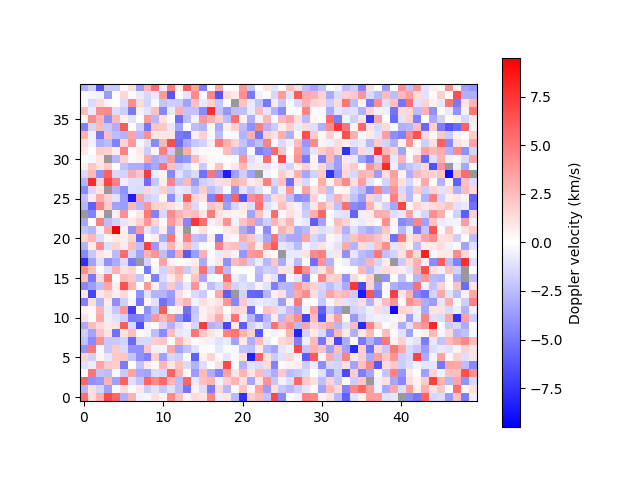```<matplotlib.image.AxesImage object at 0x7f7b37ea19a0>
```

Notice that pixels with missing data (NaN) are shown in grey.

By default, the velocity data are assumed to have units km/s. If your data are not in km/s, you must either 1) rescale the array such that it is in km/s, 2) attach an astropy unit to the array to override the default, or 3) pass an astropy unit to the `unit` parameter to override the default. For example, we can change from km/s to m/s,

```import astropy.units as u

plot_map(arr * 1000 * u.m / u.s)
```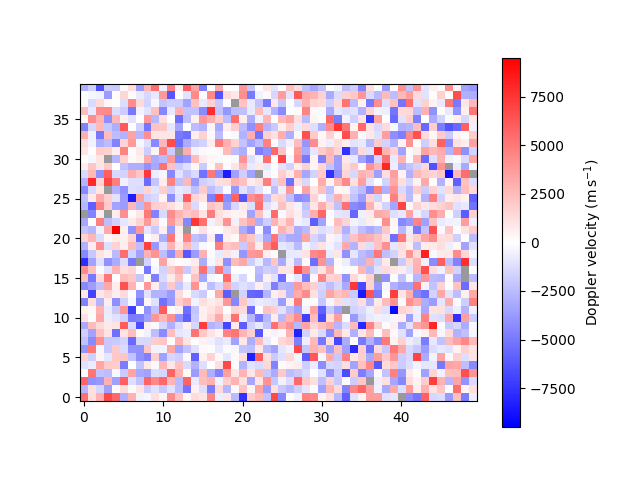```<matplotlib.image.AxesImage object at 0x7f7b37fc4f40>
```

A spatial resolution with units can be specified for each axis.

```plot_map(arr, resolution=(0.5 * u.km, 0.6 * u.Mm), offset=(-25, -20))
```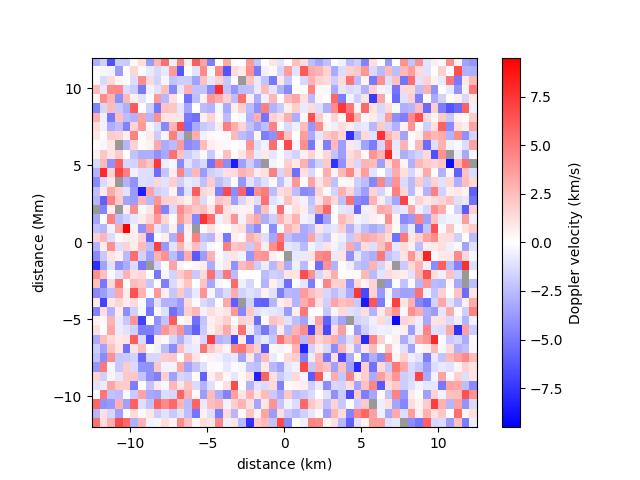```<matplotlib.image.AxesImage object at 0x7f7b5edda3a0>
```

A narrower range of velocities to be plotted can be requested with the `vmin` and `vmax` parameters. Classifications outside of the range will appear saturated. Providing only one of `vmin` and `vmax` with set the other such that zero is the midpoint.

```plot_map(arr, vmax=4)
```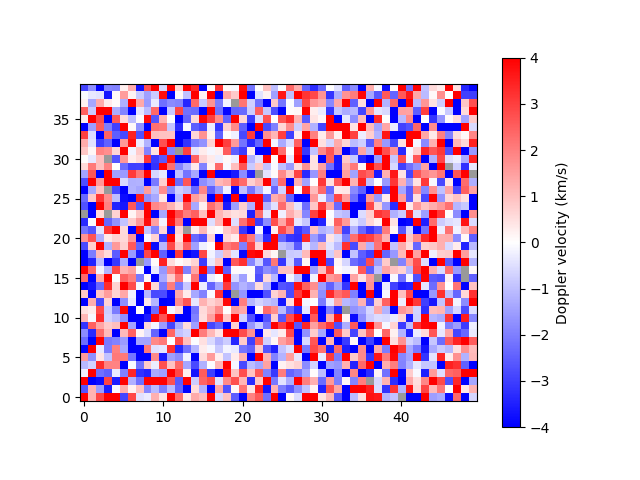```<matplotlib.image.AxesImage object at 0x7f7b37db8fd0>
```

A mask can be applied to the velocity array to isolate a region of interest. This functionally is useful if, for example, data only exist for a circular region and you want to distinguish between the pixels that are out of bounds and the data that were not successfully fitted.

```from mcalf.utils.mask import genmask

```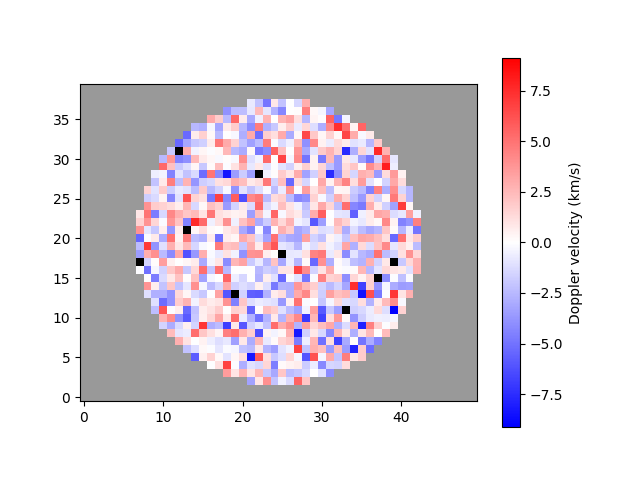```<matplotlib.image.AxesImage object at 0x7f7b3c0d4250>
```

Notice how data out of bounds are grey, while data which were not fitted successfully are now black.

A region of interest, typically the umbra of a sunspot, can be outlined by passing a different mask.

```umbra_mask = genmask(50, 40, 5, 5, 5)

```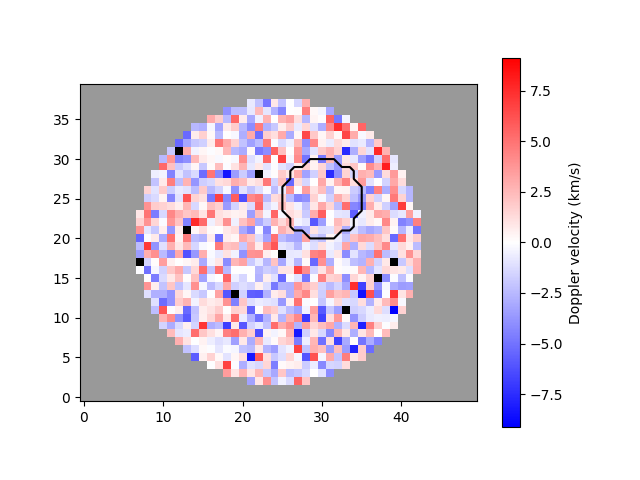```<matplotlib.image.AxesImage object at 0x7f7b3fcf69a0>
```

The plot_map function integrates well with matplotlib, allowing extensive flexibility.

```import matplotlib.pyplot as plt

fig, ax = plt.subplots(1, 2, constrained_layout=True)

plot_map(arr[:, :25], vmax=6, ax=ax, show_colorbar=False)
im = plot_map(arr[:, 25:], vmax=6, ax=ax, show_colorbar=False)

fig.colorbar(im, ax=ax, location='bottom', label='velocity (km/s)')

ax.set_title('first half')
ax.set_title('second half')

plt.show()
```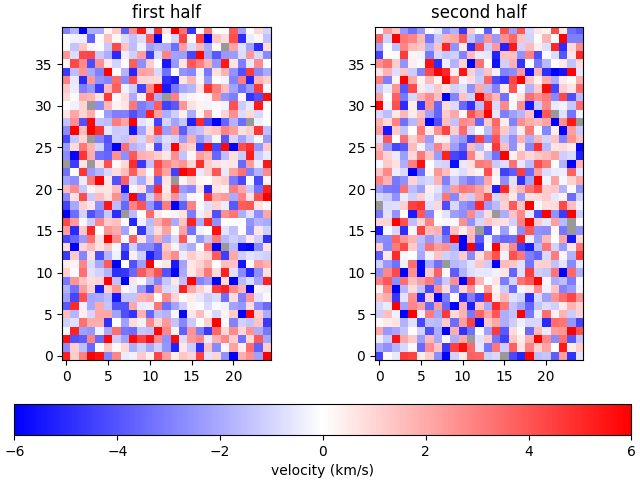Total running time of the script: ( 0 minutes 1.636 seconds)

Gallery generated by Sphinx-Gallery